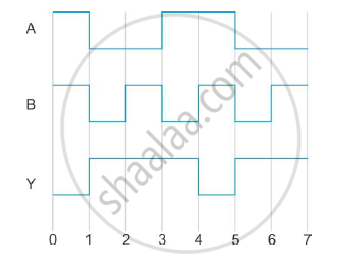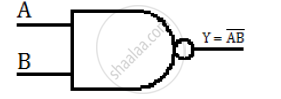# Dentify the Gate, Write Its Truth Table and Draw Its Logic Symbol. - Physics

The following figure shows the input waveforms (A, B) and the output waveform (Y) of a gate. Identify the gate, write its truth table and draw its logic symbol.#### Solution

The truth table for the gate can be obtained from the waveforms (from t = 1) as

 A B Y=bar(AB) 0 0 1 0 1 1 1 0 1 1 1 0

Therefore, from the truth table, we can say that the gate is a NAND gate. The logic symbol for the NAND gate isConcept: Digital Electronics and Logic Gates
Is there an error in this question or solution?
2015-2016 (March) All India Set 3 N

Share